# MyBatis动态SQL（认真看看， 以后写SQL就爽多了）

cnblogs.com/homejim/p/9909657.html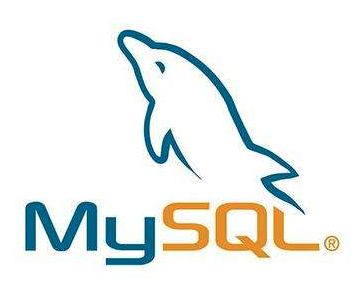# 0 一起来学习 mybatis

MyBatis 令人喜欢的一大特性就是动态 SQL。 在使用 JDBC 的过程中， 根据条件进行 SQL 的拼接是很麻烦且很容易出错的。 MyBatis 动态 SQL 的出现， 解决了这个麻烦。

MyBatis通过 OGNL 来进行动态 SQL 的使用的。

if 判断语句 单条件分支
choose(when、otherwise) 相当于 Java 中的 if else 多条件分支
trim(where、set) 辅助元素 用于处理 SQL 拼接问题
foreach 循环语句 批量插入， 更新， 查询时经常用到
bind 创建一个变量， 并绑定到上下文中 用于兼容不同的数据库， 防止 SQL 注入等

# 1 数据准备

``````DROP TABLE IF EXISTS `student`;CREATE TABLE `student` (  `student_id` int(10) unsigned NOT NULL AUTO_INCREMENT COMMENT '编号',  `name` varchar(20) DEFAULT NULL COMMENT '姓名',  `phone` varchar(20) DEFAULT NULL COMMENT '电话',  `email` varchar(50) DEFAULT NULL COMMENT '邮箱',  `sex` tinyint(4) DEFAULT NULL COMMENT '性别',  `locked` tinyint(4) DEFAULT NULL COMMENT '状态(0:正常,1:锁定)',  `gmt_created` datetime DEFAULT CURRENT_TIMESTAMP COMMENT '存入数据库的时间',  `gmt_modified` datetime DEFAULT CURRENT_TIMESTAMP ON UPDATE CURRENT_TIMESTAMP COMMENT '修改的时间',  `delete` int(11) DEFAULT NULL,
PRIMARY KEY (`student_id`)
) ENGINE=InnoDB AUTO_INCREMENT=7 DEFAULT CHARSET=utf8mb4 COLLATE=utf8mb4_0900_ai_ci COMMENT='学生表';``````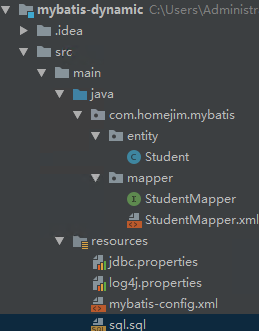# 2 if 标签

if 标签是我们最常使用的。 在查询、删除、更新的时候很可能会使用到。 必须结合 test 属性联合使用。

## 2.1 在 WHERE 条件中使用 if 标签

### 2.1.1 查询条件

1. 当只输入用户名时， 使用用户名进行模糊检索；

2. 当只输入性别时， 使用性别进行完全匹配

3. 当用户名和性别都存在时， 用这两个条件进行查询匹配查询

### 2.1.2 动态 SQL

``````    /**     * 根据输入的学生信息进行条件检索     * 1. 当只输入用户名时， 使用用户名进行模糊检索；     * 2. 当只输入邮箱时， 使用性别进行完全匹配     * 3. 当用户名和性别都存在时， 用这两个条件进行查询匹配的用     * @param student     * @return     */
List<Student> selectByStudentSelective(Student student);``````

``````  <select id="selectByStudentSelective" resultMap="BaseResultMap" parameterType="com.homejim.mybatis.entity.Student">    select
<include refid="Base_Column_List" />    from student    where 1=1
<if test="name != null and name !=''">      and name like concat('%', #{name}, '%')
</if>
<if test="sex != null">      and sex=#{sex}
</if>
</select>``````

``````    <if test="name != null and name !=''">      and name like concat('%', #{name}, '%')
</if>
<if test="sex != null">      and sex=#{sex}
</if>``````

### 2.1.3 测试

``````      @Test    public void selectByStudent() {
SqlSession sqlSession = null;
sqlSession = sqlSessionFactory.openSession();
StudentMapper studentMapper = sqlSession.getMapper(StudentMapper.class);
Student search = new Student();
search.setName("明");
System.out.println("只有名字时的查询");
List<Student> studentsByName = studentMapper.selectByStudentSelective(search);        for (int i = 0; i < studentsByName.size(); i++) {
System.out.println(ToStringBuilder.reflectionToString(studentsByName.get(i), ToStringStyle.MULTI_LINE_STYLE));
}
search.setName(null);
search.setSex((byte) 1);
System.out.println("只有性别时的查询");
List<Student> studentsBySex = studentMapper.selectByStudentSelective(search);        for (int i = 0; i < studentsBySex.size(); i++) {
System.out.println(ToStringBuilder.reflectionToString(studentsBySex.get(i), ToStringStyle.MULTI_LINE_STYLE));
}
System.out.println("姓名和性别同时存在的查询");
search.setName("明");
List<Student> studentsByNameAndSex = studentMapper.selectByStudentSelective(search);        for (int i = 0; i < studentsByNameAndSex.size(); i++) {
System.out.println(ToStringBuilder.reflectionToString(studentsByNameAndSex.get(i), ToStringStyle.MULTI_LINE_STYLE));
}
``````       sqlSession.commit();
sqlSession.close();
}``````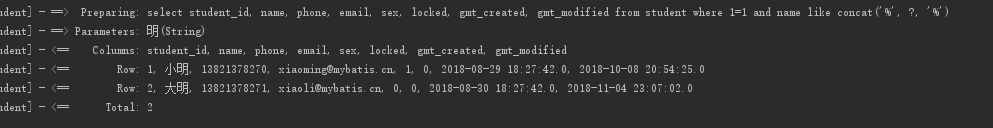``where 1=1 and name like concat('%', ?, '%') ``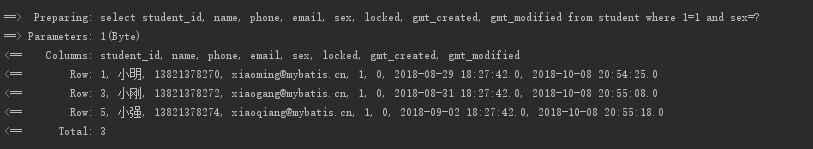`` where 1=1 and sex=? ``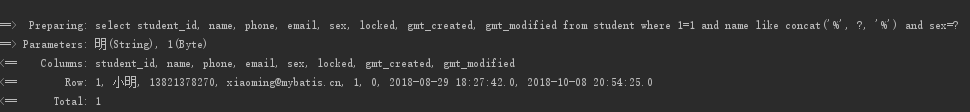``where 1=1 and name like concat('%', ?, '%') and sex=? ``

## 2.2 在 UPDATE 更新列中使用 if 标签

### 2.2.1 动态 SQL

``````    /**     * 更新非空属性     */
int updateByPrimaryKeySelective(Student record);``````

``````  <update id="updateByPrimaryKeySelective" parameterType="com.homejim.mybatis.entity.Student">
update student
<set>
<if test="name != null">
`name` = #{name,jdbcType=VARCHAR},
</if>
<if test="phone != null">
phone = #{phone,jdbcType=VARCHAR},
</if>
<if test="email != null">
email = #{email,jdbcType=VARCHAR},
</if>
<if test="sex != null">
sex = #{sex,jdbcType=TINYINT},
</if>
<if test="locked != null">
locked = #{locked,jdbcType=TINYINT},
</if>
<if test="gmtCreated != null">
gmt_created = #{gmtCreated,jdbcType=TIMESTAMP},
</if>
<if test="gmtModified != null">
gmt_modified = #{gmtModified,jdbcType=TIMESTAMP},
</if>
</set>    where student_id = #{studentId,jdbcType=INTEGER}``````

### 2.2.3 测试

``````    @Test
public void updateByStudentSelective() {
SqlSession sqlSession = null;
sqlSession = sqlSessionFactory.openSession();
StudentMapper studentMapper = sqlSession.getMapper(StudentMapper.class);
Student student = new Student();
student.setStudentId(1);
student.setName("明明");
student.setPhone("13838438888");
System.out.println(studentMapper.updateByPrimaryKeySelective(student));
``````       sqlSession.commit();
sqlSession.close();
}``````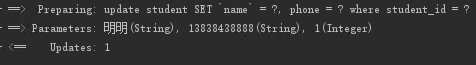## 2.3 在 INSERT 动态插入中使用 if 标签

### 2.3.2 动态SQL

``````    /**     * 非空字段才进行插入     */
int insertSelective(Student record);``````

``````<insert id="insertSelective" parameterType="com.homejim.mybatis.entity.Student">
insert into student
<trim prefix="(" suffix=")" suffixOverrides=",">
<if test="studentId != null">
student_id,
</if>
<if test="name != null">
`name`,
</if>
<if test="phone != null">
phone,
</if>
<if test="email != null">
email,
</if>
<if test="sex != null">
sex,
</if>
<if test="locked != null">
locked,
</if>
<if test="gmtCreated != null">
gmt_created,
</if>
<if test="gmtModified != null">
gmt_modified,
</if>
</trim>
<trim prefix="values (" suffix=")" suffixOverrides=",">
<if test="studentId != null">        #{studentId,jdbcType=INTEGER},
</if>
<if test="name != null">        #{name,jdbcType=VARCHAR},
</if>
<if test="phone != null">        #{phone,jdbcType=VARCHAR},
</if>
<if test="email != null">        #{email,jdbcType=VARCHAR},
</if>
<if test="sex != null">        #{sex,jdbcType=TINYINT},
</if>
<if test="locked != null">        #{locked,jdbcType=TINYINT},
</if>
<if test="gmtCreated != null">        #{gmtCreated,jdbcType=TIMESTAMP},
</if>
<if test="gmtModified != null">        #{gmtModified,jdbcType=TIMESTAMP},
</if>
</trim>
</insert>``````

### 2.3.3 测试

``````    @Test
public void insertByStudentSelective() {
SqlSession sqlSession = null;
sqlSession = sqlSessionFactory.openSession();
StudentMapper studentMapper = sqlSession.getMapper(StudentMapper.class);
Student student = new Student();
student.setName("小飞机");
student.setPhone("13838438899");
student.setEmail("xiaofeiji@qq.com");
student.setLocked((byte) 0);
System.out.println(studentMapper.insertSelective(student));
``````       sqlSession.commit();
sqlSession.close();
}``````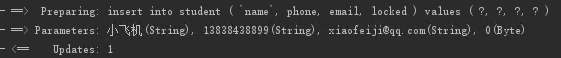SQL 中， 只有非空的字段才进行了插入。

# 3 choose 标签

choose when otherwise 标签可以帮我们实现 if else 的逻辑。

## 3.1 查询条件

• 当 studen_id 有值时， 使用 studen_id 进行查询；

• 当 studen_id 没有值时， 使用 name 进行查询；

• 否则返回空

## 3.2 动态SQL

``````    /**     * - 当 studen_id 有值时， 使用 studen_id 进行查询；     * - 当 studen_id 没有值时， 使用 name 进行查询；     * - 否则返回空     */
Student selectByIdOrName(Student record);``````

``````  <select id="selectByIdOrName" resultMap="BaseResultMap" parameterType="com.homejim.mybatis.entity.Student">    select
<include refid="Base_Column_List" />    from student    where 1=1
<choose>
<when test="studentId != null">        and student_id=#{studentId}
</when>
<when test="name != null and name != ''">        and name=#{name}
</when>
<otherwise>        and 1=2
</otherwise>
</choose>
</select>``````

## 3.3 测试

`````` @Test    public void selectByIdOrName() {
SqlSession sqlSession = null;
sqlSession = sqlSessionFactory.openSession();
StudentMapper studentMapper = sqlSession.getMapper(StudentMapper.class);
Student student = new Student();
student.setName("小飞机");
student.setStudentId(1);
Student studentById = studentMapper.selectByIdOrName(student);
System.out.println("有 ID 则根据 ID 获取");
System.out.println(ToStringBuilder.reflectionToString(studentById, ToStringStyle.MULTI_LINE_STYLE));
student.setStudentId(null);
Student studentByName = studentMapper.selectByIdOrName(student);
System.out.println("没有 ID 则根据 name 获取");
System.out.println(ToStringBuilder.reflectionToString(studentByName, ToStringStyle.MULTI_LINE_STYLE));
student.setName(null);
Student studentNull = studentMapper.selectByIdOrName(student);
System.out.println("没有 ID 和 name, 返回 null");
Assert.assertNull(studentNull);
``````       sqlSession.commit();
sqlSession.close();
}``````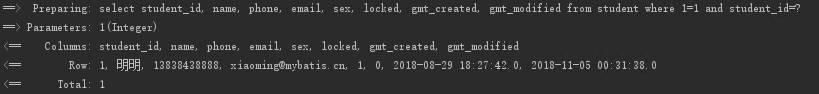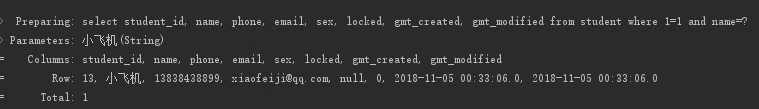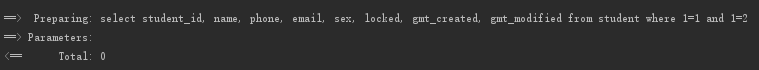# 4 trim(set、where)

## 4.1 where

### 4.1.1 查询条件

1. 当只输入用户名时， 使用用户名进行模糊检索；

2. 当只输入性别时， 使用性别进行完全匹配

3. 当用户名和性别都存在时， 用这两个条件进行查询匹配查询

## 4.1.2 动态 SQL

• 当条件都不满足时： 此时 SQL 中应该要不能有 where ， 否则导致出错

• 当 if 有条件满足时： SQL 中需要有 where， 且第一个成立的 if 标签下的 and | or 等要去掉

``````    /**     * 根据输入的学生信息进行条件检索     * 1. 当只输入用户名时， 使用用户名进行模糊检索；     * 2. 当只输入邮箱时， 使用性别进行完全匹配     * 3. 当用户名和性别都存在时， 用这两个条件进行查询匹配的用     */
List<Student> selectByStudentSelectiveWhereTag(Student student);``````

``````  <select id="selectByStudentSelectiveWhereTag" resultMap="BaseResultMap" parameterType="com.homejim.mybatis.entity.Student">    select
<include refid="Base_Column_List" />    from student
<where>
<if test="name != null and name !=''">      and name like concat('%', #{name}, '%')
</if>
<if test="sex != null">      and sex=#{sex}
</if>
</where>
</select>``````

## 4.1.3 测试

``````
@Test    public void selectByStudentWhereTag() {
SqlSession sqlSession = null;
sqlSession = sqlSessionFactory.openSession();
StudentMapper studentMapper = sqlSession.getMapper(StudentMapper.class);
Student search = new Student();
search.setName("明");
System.out.println("只有名字时的查询");
List<Student> studentsByName = studentMapper.selectByStudentSelectiveWhereTag(search);        for (int i = 0; i < studentsByName.size(); i++) {
System.out.println(ToStringBuilder.reflectionToString(studentsByName.get(i), ToStringStyle.MULTI_LINE_STYLE));
}

search.setSex((byte) 1);
System.out.println("姓名和性别同时存在的查询");
List<Student> studentsBySex = studentMapper.selectByStudentSelectiveWhereTag(search);        for (int i = 0; i < studentsBySex.size(); i++) {
System.out.println(ToStringBuilder.reflectionToString(studentsBySex.get(i), ToStringStyle.MULTI_LINE_STYLE));
}
System.out.println("姓名和性别都不存在时查询");
search.setName(null);
search.setSex(null);
List<Student> studentsByNameAndSex = studentMapper.selectByStudentSelectiveWhereTag(search);        for (int i = 0; i < studentsByNameAndSex.size(); i++) {
System.out.println(ToStringBuilder.reflectionToString(studentsByNameAndSex.get(i), ToStringStyle.MULTI_LINE_STYLE));
}
``````       sqlSession.commit();
sqlSession.close();
}``````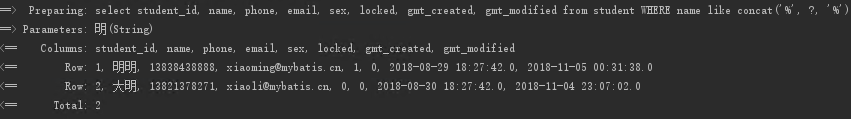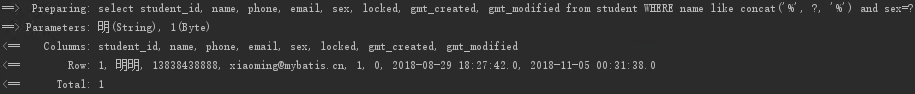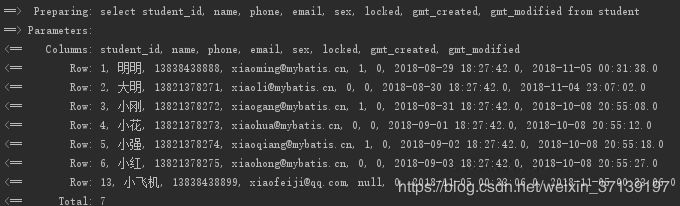## 4.2 set

set 标签也类似， 在 [2.2 在 UPDATE 更新列中使用 if 标签] 中， 如果我们的方法 `updateByPrimaryKeySelective` 没有使用

## 4.3 trim

set 和 where 其实都是 trim 标签的一种类型， 该两种功能都可以使用 trim 标签进行实现。

### 4.3.1 trim 来表示 where

``<trim prefix="where" prefixOverrides="AND |OR"></trim>``

### 4.3.2 trim 来表示 set

``<trim prefix="SET" suffixOverrides=","></trim>``

### 4.3.3 trim 的几个属性

• prefix: 当 trim 元素包含有内容时， 增加 prefix 所指定的前缀

• prefixOverrides: 当 trim 元素包含有内容时， 去除 prefixOverrides 指定的 前缀

• suffix: 当 trim 元素包含有内容时， 增加 suffix 所指定的后缀

• suffixOverrides： 当 trim 元素包含有内容时， 去除 suffixOverrides 指定的后缀

# 5 foreach 标签

foreach 标签可以对数组， Map 或实现 Iterable 接口。

foreach 中有以下几个属性

• collection: 必填， 集合/数组/Map的名称.

• item: 变量名。 即从迭代的对象中取出的每一个值

• index: 索引的属性名。 当迭代的对象为 Map 时， 该值为 Map 中的 Key.

• open: 循环开头的字符串

• close: 循环结束的字符串

• separator: 每次循环的分隔符

1. 只有一个数组参数或集合参数

2. 多参数

3. 参数是Map

4. 参数是对象

## 5.1 在 where 中使用 foreach

### 5.1.2 动态 SQL

``````    /**     * 获取 id 集合中的用户信息     * @param ids     * @return     */
List<Student> selectByStudentIdList(List<Integer> ids);``````

``````  <select id="selectByStudentIdList" resultMap="BaseResultMap">    select
<include refid="Base_Column_List" />    from student    where student_id in
<foreach collection="list" item="id" open="(" close=")" separator="," index="i">      #{id}
</foreach>
</select>``````

### 5.1.3 测试

``````    @Test    public void selectByStudentIdList() {
SqlSession sqlSession = null;
sqlSession = sqlSessionFactory.openSession();
StudentMapper studentMapper = sqlSession.getMapper(StudentMapper.class);
List<Student> students = studentMapper.selectByStudentIdList(ids);        for (int i = 0; i < students.size(); i++) {
System.out.println(ToStringBuilder.reflectionToString(students.get(i), ToStringStyle.MULTI_LINE_STYLE));
}
``````       sqlSession.commit();
sqlSession.close();
}``````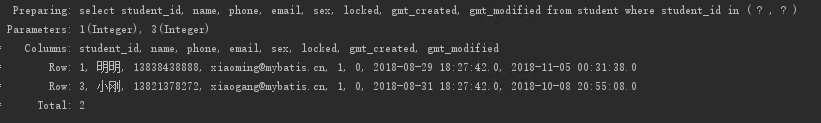## 5.2 foreach 实现批量插入

### 5.2.1 动态SQL

``````    /**     * 批量插入学生     */
int insertList(List<Student> students);``````

``````  <insert id="insertList">    insert into student(name, phone, email, sex, locked)    values    <foreach collection="list" item="student" separator=",">
(      #{student.name}, #{student.phone},#{student.email},
#{student.sex},#{student.locked}
)
</foreach>
</insert>``````

### 5.2.2 测试

``````    @Test    public void insertList() {
SqlSession sqlSession = null;
sqlSession = sqlSessionFactory.openSession();
StudentMapper studentMapper = sqlSession.getMapper(StudentMapper.class);
Student stu1 = new Student();
stu1.setName("批量01");
stu1.setPhone("13888888881");
stu1.setLocked((byte) 0);
stu1.setEmail("13888888881@138.com");
stu1.setSex((byte) 1);
Student stu2 = new Student();
stu2.setName("批量02");
stu2.setPhone("13888888882");
stu2.setLocked((byte) 0);
stu2.setEmail("13888888882@138.com");
stu2.setSex((byte) 0);
``````       System.out.println(studentMapper.insertList(students));
sqlSession.commit();
sqlSession.close();
}``````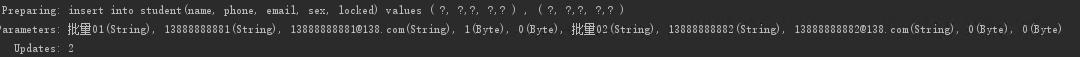# 6 bind 标签

bind 标签是通过 OGNL 表达式去定义一个上下文的变量， 这样方便我们使用。

``````<if test="name != null and name !=''">      and name like concat('%', #{name}, '%')
</if>``````

``````<if test="name != null and name !=''">
<bind name="nameLike" value="'%'+name+'%'"/>
and name like #{nameLike}</if>``````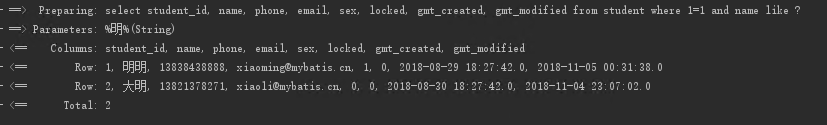# 7 代码

—————END—————#### 已有 0 条评论

欢迎您，新朋友，感谢参与互动！